# Two prizes

In a class of 15 pupils (7 boys and 8 girls), Teacher Mae is giving away a random prize. What is probability that both prizes are won by girls?

p =  0.2667

### Step-by-step explanation:Did you find an error or inaccuracy? Feel free to write us. Thank you!Tips to related online calculators
Need help to calculate sum, simplify or multiply fractions? Try our fraction calculator.
Would you like to compute count of combinations?

## Related math problems and questions:

• Ratio of countsThere are 15 boys and 13 girls in the class. What are the ratio of boys and girls?
• Boys and girlsThere are eight boys and nine girls in the class. There were six children on the trip from this class. What is the probability that left a) only boys b) just two boys
• School club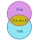Six boys and nine girls and a teacher played in the school club. What is the probability that they will point at the boy when they reproach?
• PupilsThere are 32 pupils in the classroom, and girls are two-thirds more than boys. a) How many percents are more girls than boys? Round the result to a whole percentage. b) How many boys are in the class? c) Find the ratio of boys and girls in the class. Writ
• A class IV.AIn a class there are 15 girls and 30 boys.  What fraction of the class represents the boys?
• Pizza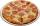A school survey found that 12 out of 15 students like pizza. If 6 students are chosen at random, what is the probability that all 6 students like pizza?
• Boys and girls 8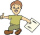In a class of 35 boys and girls that has a ratio of 2:5, 5 boys are absent and 5 boys are present. How many students are present?
• ClassIn a class are 32 pupils. Of these are 8 boys. What percentage of girls are in the class?
• Scrap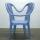From 6 products are 3 scrap. What is the probability that the random pick of 2 products have no defective product?
• In the classroomThere are 30 boys and a few girls in the class. In the six months, 28 boys and all girls benefited, which was 95% of all pupils. How many pupils are there in the classroom?
• Today in schoolThere are 9 girls and 11 boys in the class today. What is the probability that Suzan will go to the board today?
• Classroom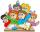In a class are 32 pupils. Boys are 8 less than girls. How many boys and girls are in the classroom?
• A class IV.CIn a class 2/5 were boys. 30 were girls. How many more girls than boys are there?
• Ski class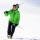Class attend 30 boys and some number of girls. Ski training was attended by 28 boys and all the girls, which was 95% of all students. How many girls attend this class? How many percent is that?
• ClassroomThere are eighty more girls in the class than boys. Boys are 40 percent and girls are 60 percent. How many are boys and how many girls?
• Drawing from a hatWhen drawing numbers from a hat from 1 to 35, we select random given numbers. What is the probability that the drawn numbers will be divisible by 8 and 2?
• Class pairs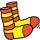In a class of 34 students, including 14 boys and 20 girls. How many couples (heterosexual, boy-girl) we can create? By what formula?